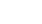# Shaking off the Rust### March 14th, 2022Difficulty: Somewhere between beginner and intermediate

Shaking off the Rust is a series of exercises with the Rust programing language. The purpose of the series is to improve both my and my dear reader’s abilities with Rust by building things. Plus, by actually building stuff, we'll learn about an array of technological concepts in the process. In this short and special installment, we’re going to estimate some digits of $\pi$.

This installment's Github repo: https://github.com/josht-jpg/shaking-off-the-rust-pi-day-special.

### Happy Pi Day

Pi, represented by the Greek letter $\pi$﻿, is one of mathematics’ favorite constants. Approximately equal to 3.141592, $\pi$﻿ is the ratio of a circle’s circumference to its diameter . $\pi$﻿ is an irrational number, meaning its digits continue infinitely with no repeating pattern.

It’s March 14th. 03/14. $\pi$﻿ day! Like true Rustaceans, we’ll celebrate by estimating some digits of $\pi$﻿ with Rust.### Getting Started

We’ll start by creating a new library in Cargo:

cargo new pi_day_special --lib
cd pi_day_special

### Estimating Digits of Pi

17th-century mathematician Gottfried Wilhelm Leibniz found this lovely infinite series:

$\frac{\pi}{4} = \frac{1}{1}-\frac{1}{3}+\frac{1}{5}-\frac{1}{7}+\frac{1}{9}...$﻿

That is, you can get $\frac{\pi}{4}$﻿ by alternatively subtracting and adding odd fractions (but you’ll have to add and subtract until the end of time) .

That’s cool. We can use this series to estimate $\pi$﻿: though we can’t compute infinitely odd fractions, we can compute the first $n$﻿, where $n$﻿ is an integer that’s not too huge:

For increasing odd numbers $k_1...k_n, \hspace{1mm} \pi \approx \frac{4}{k_1} - \frac{4}{k_2} + \frac{4}{k_3} \pm ... \pm \frac{4}{k_n}$﻿.

We’ll make a function called estimate_pi, which takes as an argument the number of decimal places of $\pi$﻿ the caller of estimate_pi wants to see. We’ll call that argument decimal_places. estimate_pi will take the approach of adding/subtracting odd fractions until the target accuracy is reached.

Here’s pseudocode for estimate_pi, which will be followed by a Rust implementation:

estimate_pi arguments {
decimal_places: number of decimal places of Pi we'd like to see
}

function estimate_pi(decimal_places)
returning the first couple digits of Pi
{
target_accuracy = 1 / 10.pow(decimal_places + 1)

result = 0
odd_divisor = 1
subtract_fraction = false

while absolute_value(current_estimate - PI) > target_accuracy {
if subtract_fraction {
result -= 4 / odd_divisor
} else {
result += 4 / odd_divisor
}
odd_divisor += 2
subtract_fraction = !subtract_fraction
}

return result
}

And repeating that in Rust:

//  lib.rs

use std::f64::consts::PI;

fn estimate_pi(decimal_places: u32) -> f64 {
assert!(
decimal_places < 9,
"It's not worth freezing your computer over this, my friend."
);

let target_accuracy = 1. / 10u32.pow(decimal_places + 1) as f64;
let is_accurate_enough =
|current_estimate: f64| (current_estimate - PI).abs() < target_accuracy;

let mut result = 0.;
let mut odd_divisor = 1;
let mut subtract_fraction = false;

while !is_accurate_enough(result) {
result += if subtract_fraction {
-4. / odd_divisor as f64
} else {
4. / odd_divisor as f64
};
odd_divisor += 2;
subtract_fraction = !subtract_fraction;
}

result
}

Nice.

Let’s discuss the parts of that Rust code that are possibly interesting and novel to you (as always, if I miss something you’re curious about, don’t hesitate to email me: joshtaylor361@gmail.com).

• Check out 10u32 in the line
let target_accuracy = 1. / 10u32.pow(decimal_places + 1) as f64;
The u32 type suffix is a way of specifying that we want the numeric literal 10 to be of type u32. If we don’t make this specification, we’ll run into this error: can't call method pow on ambiguous numeric type {integer}. Don’t want that.

And if we specify that 10 is a u8, we’ll get this error at runtime: panicked at 'attempt to multiply with overflow'. Definitely don’t want that.

• let is_accurate_enough = |current_estimate: f64| (current_estimate - PI).abs() < target_accuracy;
This is a closure. In Rust, closures are anonymous functions that can capture their environment . That is, a closure is a function that's able to access data from its surrounding environment : in our code, is_accurate_enough uses target_accuracy without taking it as an argument; it captures target_accuracy.

Closures are an integral part of the Rust programmer’s toolkit, and there’s a lot more to say about them. If you want to learn more, I recommend:

• If you come from a C background, this little diddy may have caused you to do a double take:
result += if subtract_fraction {
-4. / odd_divisor as f64
} else {
4. / odd_divisor as f64
};

Unlike C, Rust is an expression language . Expressions are a combination values and functions that work together to produce a new value. Most stuff you see in Rust is an expression .

In C, if is a statement, meaning it doesn’t produce a value. But in Rust, if is an expression: it produces a value. So we can add the value produced by our if block to result. I love Rust 🦀.

We’ll finish up with a test:

//  lib.rs

/*...*/

#[cfg(test)]
mod tests {
use super::*;

#[test]
fn estimation_test() {
assert!((PI - estimate_pi(2)).abs() < 0.001);
assert!((PI - estimate_pi(3)).abs() < 0.0001);
assert!((PI - estimate_pi(5)).abs() < 0.000001);
assert!((PI - estimate_pi(7)).abs() < 0.00000001)
}
}

If that passes, you have celebrated $\pi$﻿ day like a true Rustacean. We salute you.

### References

 - What is Pi? piday.org

## Support Me

Creating and running Shaking off the Rust is one of the most fulfilling things I do. But it's exhausting. By supporting me, even if it's just a dollar, you'll allow me to put more time into building this series. I really appreciate any support.

The only way to support me right now is by sponsoring me on Github. I'll probably also set up Patreon and Donorbox pages soon.

Thank you so much!